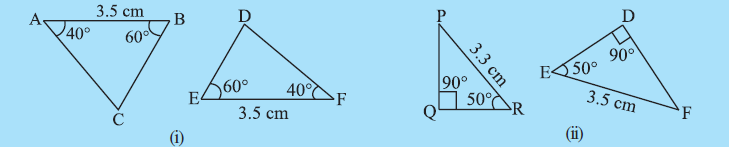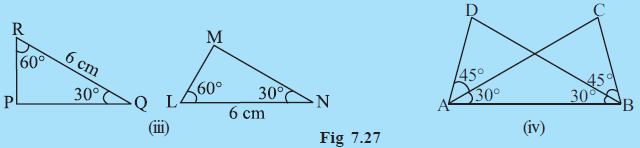Q

# In Fig 7.27, measures of some parts are indicated. By applying ASA congruence rule, state which pairs of triangles are congruent. In case of congruence, write the result in symoblic form.

3. In Fig 7.27, measures of some parts are indicated. By applying ASA congruence rule, state which pairs triangles are congruent. In case of congruence, write the result in symoblic form.Views

i) in  and

AB = FE = 3.5 cm

So by ASA congruency rule, both triangles are congruent.i.e.

ii) in  and

But,

So, given triangles are not congruent.

iii) in  and

RQ = LN = 6 cm

So by ASA congruency rule, both triangles are congruent.i.e.

.

iv) in  and

AB = BA (common side)

So by ASA congruency rule, both triangles are congruent.i.e.

Exams
Articles
Questions